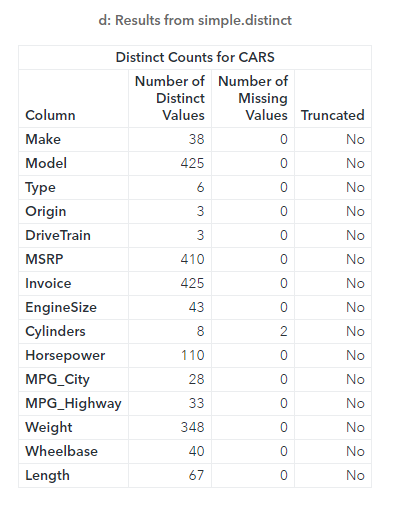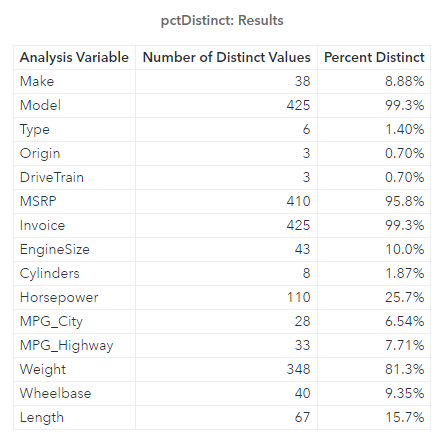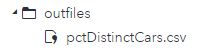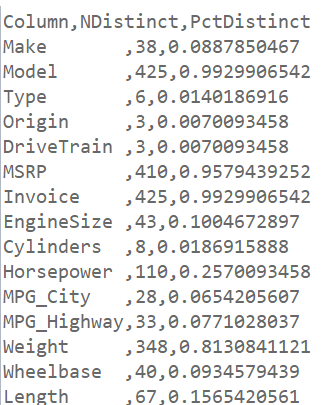In my previous post CAS-Action! Simply Distinct - Part 1 I reviewed using the simple.distinct CAS action to explore distinct and missing values in a distributed CAS table.

Welcome back to my SAS Users blog series CAS Action! - a series on fundamentals. I've broken the series into logical, consumable parts. If you'd like to start by learning a little more about what CAS Actions are, please see CAS Actions and Action Sets - a brief intro. Or if you'd like to see other topics in the series, see the overview page.

And now, back to the distinct action. What if we want to do more? Maybe you want to create a CSV file that documents the percentage of distinct values in each column? Let's explore some possibilities.

To complete the task I'll break it down into four steps.

## Step 1 - Find the number of rows in the CAS table

To find the number of rows in a CAS table use the simple.numRows CAS action. Let's execute the numRows action and store the results in a variable. I'll also PRINT and DESCRIBE the results to take a closer look at the output.

```proc cas; simple.numRows result=n / table={name="cars",caslib="casuser"}; describe n; print n; ...```

The results of the DESCRIBE statement show the output of the action is a dictionary with a key named numRows and an integer as the value. The PRINT statement shows the value of the dictionary, numRows=428.

Now that we have the total number of rows, we can use that number in our calculation.

## Step 2 - Find the number of distinct values in each column

Next, let's execute the distinct action and store the results in a variable named d. Then execute the PRINT statement to confirm the results.

```... simple.distinct result=d / table={name="cars",caslib="casuser"}; print d; ...```

And the resluts:The results of the distinct action are as expected. Each column with the number of distinct values.

## Step 3 - Create a calculated column that computes the percentage of distinct values

Now that we have the number of distinct values in each column, and the total number of rows in the CARS CAS table, we can calculate the total percent of distinct values in each column.

Consider the code:

```... pctDistinct=d.Distinct.compute({"PctDistinct","Percent Distinct",percent7.2}, nDistinct/n.numRows) [ , {"Column","NDistinct","PctDistinct"} ]; print pctDistinct; ...```

To add a calculated column to a result table use the compute operator. In the first argument, specify column metadata inside an array (column name, label and format). In the second argument, specify the expression. My expression nDistinct/n.numRows divides the distinct values in each column by the total number of rows in the CARS table.

After the compute operator, select specific rows and columns from the result table using bracket notation. Here I'll select all rows, and only the columns Column, nDistinct and PctDistinct.

Lastly, I used the PRINT statement to confirm the results.In the output we can see the new result table with the computed column.

## Step 4 - Save the results table as a CSV file

Lastly, let's put it all together!

I'll add the code from the pervious steps, then save the table as a CSV file using the SAVERESULTS statement with the CSV= option.

```%let outpath=/*specify output file location*/;   proc cas; * Specify the CAS table *; casTbl={name="cars", caslib="casuser"};   * Store the number of rows in the CAS table *; simple.numRows result=n / table=casTbl;   * Store the number of distinct values in each column *; simple.distinct result=d / table=casTbl;   * Calculate the percentage of distinct values in each column *; pctDistinct=d.Distinct.compute({"PctDistinct","Percent Distinct",percent7.2}, nDistinct/n.numRows) [ , {"Column","NDistinct","PctDistinct"} ];   * Save the result table as a CSV file *; saveresult pctDistinct csv="&outpath/pctDistinctCars.csv"; quit;```

In the CSV= option I specified the outpath macro variable that contains the location of output folder, and add the name of the CSV file.

After executing the code the log indicates everything ran successfully, and a CSV file was created in the specified location. Next I'll find and open the CSV file.

My CSV file is located in my outfiles folder:Then double click on the file to open it in SAS Studio:## Summary

The distinct action is a flexible and easy way to explore your data. It allows you to quickly explore your distributed CAS tables, then process and save the results in a variety of formats to fit your needs.